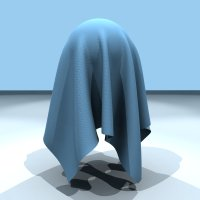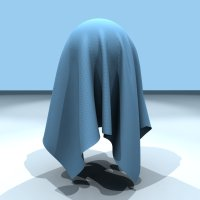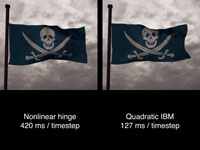Can the nature of isometric deformations be exploited to speed up simulations of materials such as cloth?

This project addresses this issue by looking at the Laplace-Beltrami operator on a surface. Applying this linear operator to the immersion of a surface gives the mean curvature normal of the surface. Under the class of isometric deformations of the surface, this operator is invariant, so it only needs to be computed once. If we consider a bending energy given as the square of mean curvature, then we see this leads to a naturally quadratic expression without any approximations.

## Publications

"Discrete Quadratic Curvature Energies"
Max Wardetzky, Miklós Bergou, David Harmon, Denis Zorin, Eitan Grinspun
Computer Aided Geometric Design 2007
[PDF]

"Discrete Quadratic Curvature Energies"
Miklós Bergou, Max Wardetzky, David Harmon, Denis Zorin, Eitan Grinspun, Eitan Grinspun (ed.)
Discrete Differential Geometry: An Applied Introduction, ACM SIGGRAPH, pp. 20-29, July 2006
[PDF]

"A Quadratic Bending Model for Inextensible Surfaces"
Miklós Bergou, Max Wardetzky, David Harmon, Denis Zorin, Eitan Grinspun
Fourth Eurographics Symposium on Geometry Processing, pp. 227-230, June 2006
[PDF]

You can download source code to compute the local and global Q matrix as described in the paper.These images show the similar qualitative behavior of full nonlinear bending (left) to quadratic bending (right) for some representative frames.These images show the results of surface fairing using Willmore flow. The algorithm used is the inexact Newton's method with the quadratic bending Hessian matrix used in the Newton solver's inner loop.